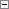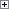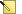A constant is the simplest type of operand used in C/AL. The value of a constant cannot be changed during the execution of the code. Constants can be defined for each of the simple data types in C/AL.Note
Numbers such as 2,147,483,647 and 999,999,999,999,999.99 cannot be entered in the C/AL editor in this form that uses commas. The commas are only used to increase the legibility when describing large numbers. If you use commas when you enter numbers in the C/AL editor, a compilation error occurs.

Boolean Constant

A Boolean constant can have either the value TRUE or FALSE.

Integer Constant

An integer constant has a value in the range -2,147,483,647 to 2,147,483,647.

Decimal Constant

A decimal constant must contain a decimal point "." (depending on your regional settings) and have at least one digit to the right of the decimal point (for example, the digit "0"). A constant of type decimal can be used to represent decimal numbers between -999,999,999,999,999.99 and 999,999,999,999,999.99 with 18 significant digits.

Date Constant

A date constant is written as six or eight digits followed by the letter "D." The date constant expressing "undefined date" is entered as "0D." The digits specify the date in the format MMDDYY or MMDDYYYY.

Time Constant

A time constant is written as six or nine digits followed by the letter "T." The "undefined time" constant is entered as "0T." The nine digits specify the time in the format HHMMSS[.XXX], that is, a 24 hour format with an optional part specifying thousandths of a second.

Text Constant

A text constant is a character string. Unique IDs are assigned to text constants so that an ID number represents a specific text constant. Examples of text constants are error messages, messages, and warnings.

Examples

The following table shows examples of different types of C/AL constants.Note
The format depends on the regional format that is set on your computer. The examples in the table correspond to a computer that has the regional format set to English (United States).

Constant Description

50000

Integer constant

-23.7

Decimal constant

122101D

Date constant (December 21, 2001)

141230T

Time constant (the time 14:12:30)

ABC

Text constant